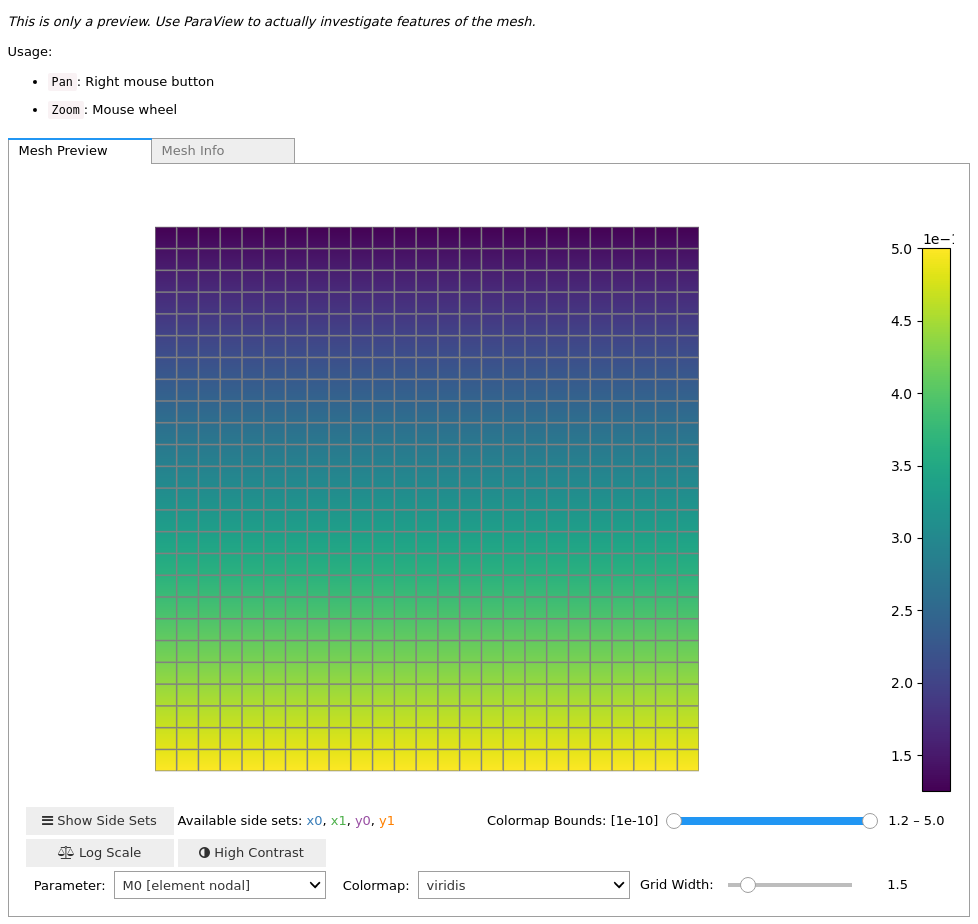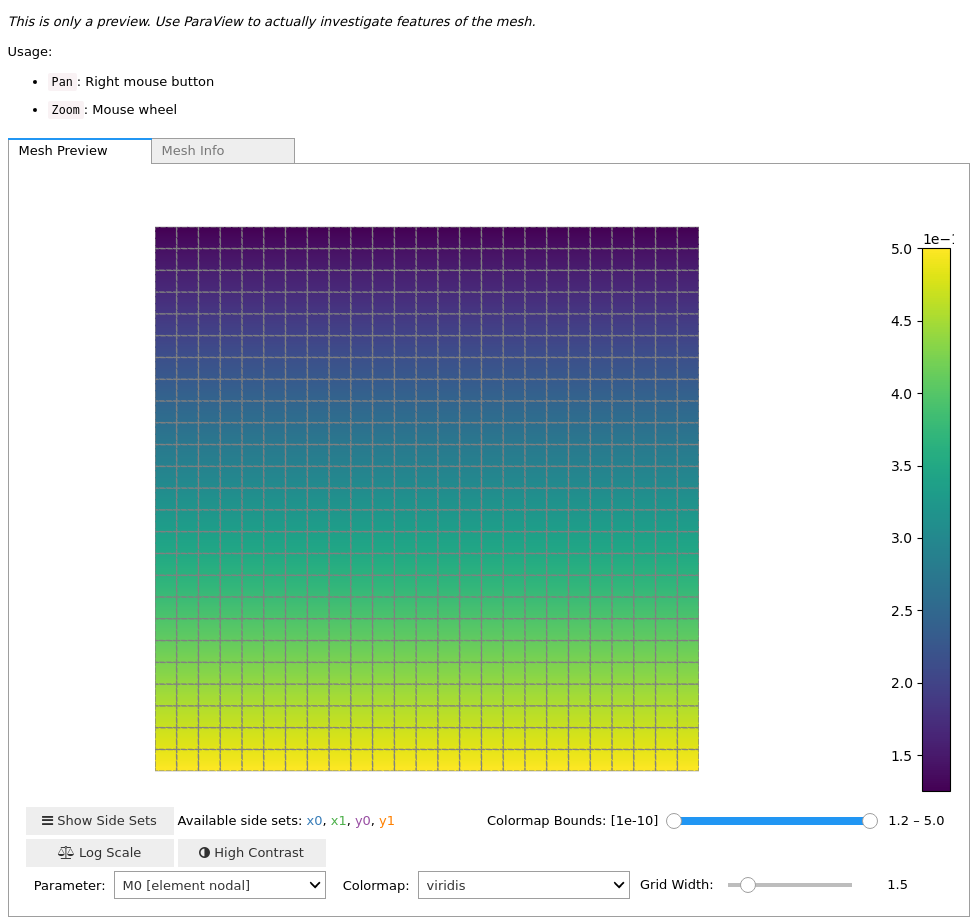Version:

This documentation is not for the latest stable Salvus version.This tutorial is presented as Python code running inside a Jupyter Notebook, the recommended way to use Salvus. To run it yourself you can copy/type each individual cell or directly download the full notebook, including all required files.# Model Interpolation

In this notebook we'll explore the concept of "model interpolation order". The spectral-element method works by approximating the dynamic field variables as Lagrange polynomials with time-varying coefficients. These polynomials are defined using the Gauss-Lobatto-Legendre (GLL) collocation points defined within each element. For a polynomial interpolation order of degree 4 (the default in Salvus), there will be 5 GLL points along each edge of a given element, which results in 25 and 125 points each for 2-D and 3-D elements, respectively. In general, a $d$-dimensional spectral element equipped with order $n$ interpolating polynomials will have $(n+1)^d$ GLL points in its interior.

While the GLL basis is the canonical method for representing the wavefield, we can also use it to represent the material parameters and spatial geometry. This tutorial shows how one can use different order (1, 2, and 4) interpolating polynomials to represent the model in Salvus. Specifically, we'll:

• Discretize a linearly-varying 2-D model onto 1st and 4th order polynomial bases
• Compare the wavefield solutions generated in each of these cases.
• Do the same in 3-D.
Copy
%matplotlib inline
%config Completer.use_jedi = False
import os
from functools import partial
from pathlib import Path

import h5py
import matplotlib.pyplot as plt
import numpy as np

import salvus.mesh.structured_grid_2D as sg2d
import salvus.mesh.structured_grid_3D as sg3d
import salvus.mesh.unstructured_mesh as um
import salvus.toolbox.toolbox as st
from salvus.flow import api
from salvus.flow import simple_config as sc

SALVUS_FLOW_SITE_NAME = os.environ.get("SITE_NAME", "local")
ranks = 2

## Setting up the models

First we set up a simple function to return basic meshes for testing in both 2 and 3 dimensions. The size of the mesh is small as these examples will only run for a trivial duration, and are just meant to be used to explore the possible parameterizations. We'll also set up a constant density throughout the domain.

n = 25
x = 1.0
rho = 1000.0
mesh = sg2d.StructuredGrid2D.rectangle(
nelem_x=n, nelem_y=n, max_x=x, max_y=x
).get_unstructured_mesh()
mesh.find_side_sets()

Now we copy the mesh skeleton we created above into two separate meshes, each of which we'll set to a different model interpolation order. For brevity we'll use here orders 1 and 4 (order 2 is also supported and the workflow is identical).

mesh_order_1 = mesh.copy()
mesh_order_1.change_tensor_order(1)

mesh_order_4 = mesh.copy()
mesh_order_4.change_tensor_order(4)

Now we'll copy the coordinate arrays for use with the interpolation function we will write below. Note that we randomly choose which axis to interpolate over.

axis = np.random.choice([0, 1])
coords_order_1 = mesh_order_1.get_element_nodes()[:, :, axis].flatten()
coords_order_4 = mesh_order_4.get_element_nodes()[:, :, axis].flatten()

The function below will linearly interpolate a range of model parameters over the coordinate range of a mesh.

def li(x: np.ndarray, min_val, max_val) -> np.ndarray:
"""Linearly interpolate a parameter over a coordinate array.

Parameters
----------
x : np.ndarray
Flattened coordinate array (either x or y).
min_val : float
Value of the parameter at the minimum coordinate.
max_val : float
Value of the parameter at the maximum coordinate.

Returns
-------
np.ndarray
An array of values linearly interpolated over the coordinate range.

"""
m = (max_val - min_val) / (np.max(x) - np.min(x))
return m * x + min_val

We'll use the linear $M0$ and $M1$ to parameterize our acoustic domain. For more information on different supported parameterizations in Salvus, please refer to the relevant tutorial.

vp0, vp1 = 1000, 2000
m10 = m11 = 1 / rho
m00 = m10 / vp0 ** 2
m01 = m11 / vp1 ** 2

Now we interpolate and attach the desired parameter to our 1st order mesh...

m0 = (np.apply_along_axis(li, 0, coords_order_1, m00, m01)).reshape(
mesh_order_1.nelem, mesh_order_1.nodes_per_element
) * 0.5

m1 = (np.apply_along_axis(li, 0, coords_order_1, m10, m11)).reshape(
mesh_order_1.nelem, mesh_order_1.nodes_per_element
) * 0.5

mesh_order_1.attach_field("M0", m0)
mesh_order_1.attach_field("M1", m1)
mesh_order_1.attach_field("fluid", np.ones(mesh.nelem))
mesh_order_1<salvus.mesh.unstructured_mesh.UnstructuredMesh at 0x7faf917a3590>

...and our 4th order mesh.

m0 = (np.apply_along_axis(li, 0, coords_order_4, m00, m01)).reshape(
mesh_order_4.nelem, mesh_order_4.nodes_per_element
) * 0.5

m1 = (np.apply_along_axis(li, 0, coords_order_4, m10, m11)).reshape(
mesh_order_4.nelem, mesh_order_4.nodes_per_element
) * 0.5

mesh_order_4.attach_field("M0", m0)
mesh_order_4.attach_field("M1", m1)
mesh_order_4.attach_field("fluid", np.ones(mesh.nelem))

mesh_order_4<salvus.mesh.unstructured_mesh.UnstructuredMesh at 0x7faf917a3850>

Note here how both meshes end up do looking the same! This is because, no matter which interpolation order we choose, Salvus must regardless interpolate the material parameters from the grid defining the model interpolation to the grid defining the wavefield interpolation. This interpolation itself is a bilinear operation which, for the linear function we've chosen to interpolate, will return the same results (to numerical precision) as simply doing the interpolation oneself. Nevertheless, this is our point here as we want to show that the generated solutions are also the same. For an application where higher-order models make a significant different in the solution, check our the Marmousi tutorial in the "exploration" section.

## Setting up a simulation (order 1)

Now we'll set up some sources and receivers as is standard. Here we're mocking a "cross-hole" setup, where we have a source in one borehole and a line of receivers in another.

# Source in the left borehole.
src = sc.source.cartesian.ScalarPoint2D(
x=0.1, y=0.5, f=1, source_time_function=sc.stf.Ricker(center_frequency=1e4)
)

# String of receivers in the right borehole.
y=np.linspace(0, 1, 101),
side_set_name="x1",
station_code="xx",
fields=["phi"],
offset=-0.1,
)

w1 = sc.simulation.Waveform(mesh=mesh_order_1, sources=src, receivers=recs)

Now we just need to set a few more parameters and we're good to go.

# Output receivers in simplified HDF5 format.
w1.output.point_data.format = "hdf5"

# Run the simulation long enough that wave hit the receivers.
w1.physics.wave_equation.end_time_in_seconds = 1e-3

# Output the state variable at all time steps (for comparison).
w1.output.volume_data.format = "hdf5"
w1.output.volume_data.fields = ["phi"]
w1.output.volume_data.filename = "output.h5"
w1.output.volume_data.sampling_interval_in_time_steps = 1

# Validate and plot.
w1.validate()
w1